GRE Subject Test: Math : Change of Base

Example Questions

Example Question #1 : Change Of Base

Evaluate: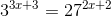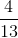Explanation:

In order to evaluate the unknown variable, it is necessary to change the base. Looking at the right side of the equation, 27 is equivalent to three cubed.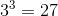Therefore, converting the right side of the equation to a base of 3 will allow setting both the left and right side of the exponential terms equal to each other.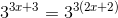Log both sides to drop the exponents by log properties, and divide the log based 3 on both sides to cancel this term.

Solve for x.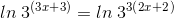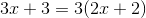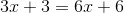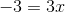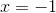Example Question #1 : Change Of Base

Evaluate the following logarithm: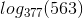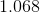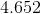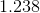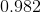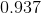Explanation:

The simplest way to evaluate a logarithm that doesn't have base 10 is with change of base formula: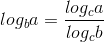So we have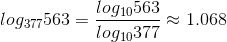Example Question #3 : Change Of Base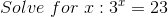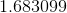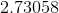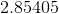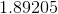Explanation:

In order to solve a logarithm, we must first rewrite it in log form: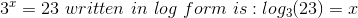To solve for x, we must use the Change of Base: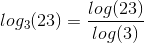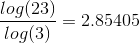This means that: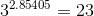All GRE Subject Test: Math Resources